Two wires carry current I1 = 47 A and I2 = 29 A in the opposite directions parallel to the x-axis at y1 = 9 cm and y2 = 15 cm. Where on the

Question

Two wires carry current I1 = 47 A and I2 = 29 A in the opposite directions parallel to the x-axis at y1 = 9 cm and y2 = 15 cm. Where on the y-axis (in cm) is the magnetic field zero?

in progress 0
5 months 2021-09-02T15:34:50+00:00 1 Answers 0 views 0

Approximately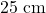, assuming that the permeability between the two wires is constant, and that the two wires are of infinite lengths.

Explanation:

Note that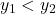, which means that the first wire (with current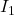) is underneath the second wire (with current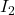.) Let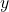denote the-coordinate (in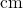) of a point of interest. The two wires partition this region into three parts:

• The region under the first wire, where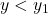;
• The region between the first and the second wire, where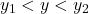; and
• The region above the second wire, where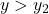.

Apply the right-hand rule to find the direction of the magnetic field in each of the three regions. Assume thatpoints to the left whilepoints to the right. Let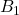and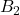denote the magnetic field due toand, respectively.

• In the region below the first wire,points out of the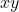-plane whilepoints into the-plane.
• In the region between the two wires, bothandpoint into the-plane.
• In the region above the second wire,points into the-plane whilepoints out of the-plane.

The (net) magnetic field on this plane would be zero only in regions whereandpoints in opposite directions. That rules out the region between the two wires.

At a distance of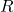away from a wire with current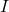and infinite length, the formula for the magnitude of the magnetic field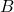due to that wire is: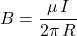,

where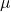is the permeability of the space between the wire and the point of interest (should be constant.) The exact value ofdoes not affect the answer to this question, as long as it is constant throughout this region.

Note that the value ofin this formula is supposed to be positive. Let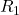and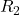denote the distance between the point of interest and the two wires, respectively.

• In the region under the first wire,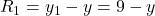, while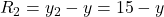.
• In the region above the second wire,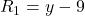, while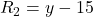.

Make sure that given the corresponding range of, these distances are all positive.

• Strength of the magnetic field due to the first wire: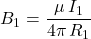.
• Strength of the magnetic field due to the second wire: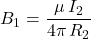.

For the net magnetic field to be zero at a certain-value, the strength of the two magnetic fields at that point should match. That is: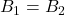.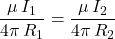.

Simplify this equation: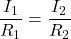.

• In the region under the first wire (where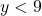,) this equation becomes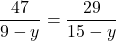.
• In the region above the second wire (where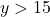,) this equation becomes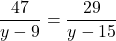.

These two equations give the same result: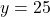. However, based on the respective assumptions on the value of, this value corresponds to the region above the second wire.